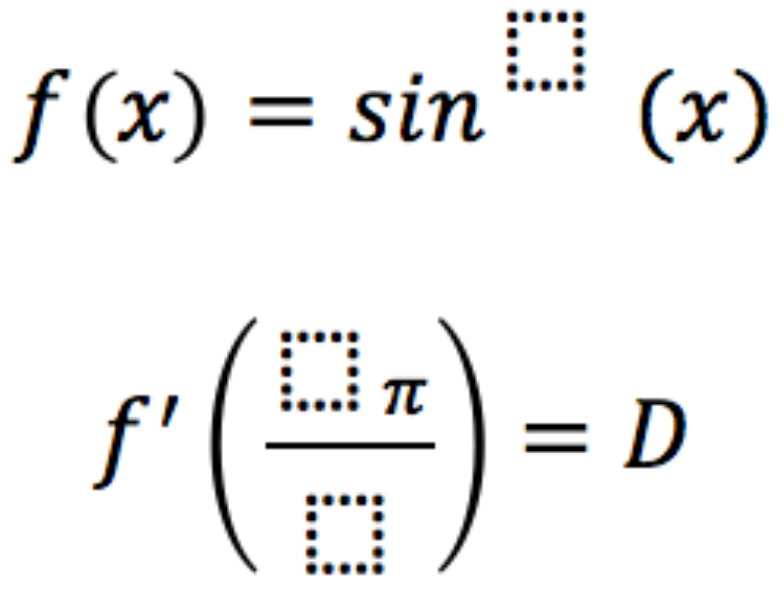# Derivative of Trig Functions 2

Directions: Fill in the boxes below using the digits 1 to 6, at most one time each, to make the largest value for D (the derivative).### Hint

What values give an output of 1 or 1/2 to the derivative of sin(x)?

sin^6(x) and f'(4pi/2)…or sin^6(x) and f'(2pi/1)

Source: Chris Luzniak

## Fundamental Theorem Of Calculus

Directions: Using the digits 0 to 9 at most one time each, place a digit …

1.The derivative of f(x)=sin^6(x) is f'(x)=6sin^5(x)*cos(x)

So f'(2pi)=0 b/c sin(2pi)=0

I believe the correct answer should be f(x)=sin^6(x) and f'(1pi/3)=1.461

•I agree, maximizing cosine will zero the sine function. Mr. H has the correct answer, to the best of my ability to judge.

•Thanks Chris and Mr. H

I believe that f'(4pi/3) = f'(pi/3) so this provides another possible solution.

2.Yes, I believe that you are correct Mr. H.